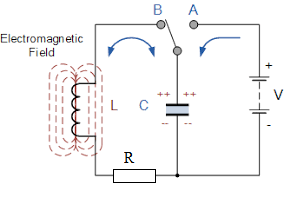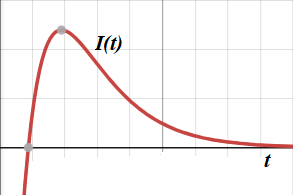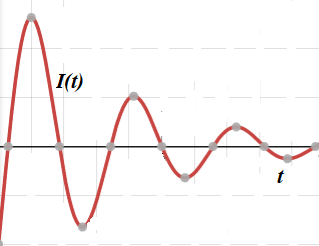## Wednesday, November 24, 2021

### RLC Circuit: UNIZOR.COM - Physics4Teens - Waves - Electronic Oscillator

Notes to a video lecture on http://www.unizor.com

RLC Circuit

The previous lecture considered a simple circuit that contained a battery, a capacitor C and an inductor L, while ignoring an internal resistance of a circuit R.
This lecture continues the exploring the oscillating characteristics of this circuit, but, in addition, we will address more practical case of the existence of an electrical resistance R in the circuit.
These circuits are called RLC-circuits.As in the previous lecture, we put an AB-switch in the A position to charge the capacitor from a battery.
Then we switch to the B position, battery is no longer a factor, and capacitor is charged.

Initially, the voltage between the plates of a capacitor is at maximum, so the electric current is at maximum as well.
As the charge goes from one plate to another through an inductor and a resistor, the voltage and the electric current are diminishing.

Assume, the electric current in the circuit is changing with time t as I(t), the voltage drop between the plates of the capacitor is VC(t), the voltage drop on the inductor is VL(t) and the voltage drop on the resistor is VR(t).

By definition of the capacitance C of a capacitor,
C = Q(t)/VC(t) or
C·VC(t) = Q(t)
where Q(t) is the charge on its plates at time t.
We prefer to deal with the electric current I(t), which is defined as amount of moving charge per unit of time, which is mathematically expressed as a derivative of a charge by time,
I(t) = dQ(t)/dt

Differentiating the above equation between voltage and charge on a capacitor, we obtain the dependency between voltage and current on a capacitor:
dVC(t)/dt = I(t) or
V'C(t) = I(t)/C

Any current that goes along a wire creates a magnetic field around this wire. Since the electric current in our inductor is variable, the magnetic field that is generated by this current is variable.

According to the Faraday's Law, the variable magnetic field going through a wire loop generates electromotive force (EMF) equal to a rate of change of the magnetic field flux.
Therefore,
VL(t) = dΦ(t)/dt or
VL(t) = Φ'(t)

In turn, magnetic flux Φ(t) going through inductor, as a function of time t, is proportional to an electric current I(t) going through its wire
Φ(t) = L·I(t)
where L is a coefficient of proportionality that depends on physical properties of the inductor called inductance of the inductor.
From this we derive the following equation that describes the relationship between VL(t) and I(t):
VL(t) = L·I'(t)

According to the Ohm's Law, the voltage drop on the resistor is
VR(t) = R·I(t)

Finally, since the circuit contains no sources of electrical energy, sum of all voltage drops should be equal to zero:
VC(t) + VL(t) + VR(t) = 0

Now we have the following system of four equations with four unknown functions:
V'C(t) = I(t)/C
VL(t) = L·I'(t)
VR(t) = R·I(t)
VC(t) + VL(t) + VR(t) = 0

Differentiating the last equation by t and substituting voltages through their expressions in terms of electric current, we obtain
L·I"(t) + R·I'(t) + I(t)/C = 0

The last equation resembles the one that describes damped mechanical oscillations of an object on an ideal spring
m·x"(t) + c·x'(t) + k·x(t) = 0
where
x(t) is a displacement of an object on a spring from the neutral position,
m is an object's mass,
c is a damping coefficient,
k is a spring's elasticity
(see lecture UNIZOR.COM - "Physics 4 Teens" - "Waves" - "Mechanical Oscillations" - "Viscosity Damping 1")

As we described in the lecture "Viscosity Damping 1", the general solution to a homogeneous linear differential equation of the second order, like the one above, can be found by finding two functions that satisfy this equation x1(t) and x2(t), called partial solutions, and describing the general solution as a linear combination of these two functions
x(t) = A·x1(t) + B·x2(t)
The coefficients A and B depend on two initial conditions x(0) and x'(0).

In this case of electronic oscillation mathematics are exactly the same, which is quite remarkable.
Let's proceed with a solution along the same path as presented in the lecture on damped mechanical oscillations.

We will seek partial solutions based on function I(t) = eγ·t.
where γ - some (generally, complex) number. The reason for this is that, substituting this function with its first and second derivatives into the differential equation, we get
I'(t) = γ·eγ·t
I"(t) = γ²·eγ·t
and the differential equation is
γ²·L·eγ·t + γ·R·eγ·t + eγ·t/C = 0

Canceling eγ·t, which never equal to zero, we get a quadratic equation for γ
γ²·L + γ·R + (1/C) = 0
with any of its solutions (generally, complex), used in function eγ·t, delivering the partial solution of the original differential equation.

Since a quadratic equation has, generally speaking, two solutions among complex numbers γ1 and γ2, we have two solutions to our original differential equations:
I1(t)=eγ1·t and I2(t)=eγ2·t.

Moreover, since our equation is a homogeneous linear differential equation of the second order, all linear combinations of the above two solutions deliver a general solution that looks like
I(t) = A·eγ1·t + B·eγ2·t
where constants A and B are defined by initial conditions on a circuit.

Switching from theory to concrete results for our differential equation presented above, our first task is to solve the quadratic equation to find γ1 and γ2
The quadratic equation for γ is
γ²·L + γ·R + (1/C) = 0

This is exactly analogous to damped mechanical oscillations (see three "Viscosity Damping" lectures in the "Mechanical Oscillations" topics of this course), where the corresponding equation looks like
γ²·m + γ·c + k = 0
(m - mass, c - damping coefficient, k - elasticity of a spring).

Solutions to this equation that describes the (generally, complex) exponent of damped mechanical oscillations are
γ1,2 = −c/(2m)±√(c/(2m))²−k/m

Substituting inductance L of an electronic oscillator for mass m of mechanical one, resistance R of an electronic oscillator for damping coefficient c of mechanical one and inverse of capacitance 1/C of an electronic oscillator for elasticity k of mechanical one, we can use all the calculations of damped mechanical oscillations to obtain corresponding results for damped oscillations of electronic oscillator.

Solutions to our quadratic equation for γ, therefore, are:
γ1,2 = −R/(2L) ±
± √(R/(2L))²−1/(L·C)

Following the same logic as in "Viscosity Damping" lectures, we consider three cases:
Δ = (R/(2L))²−1/(L·C) > 0
Δ = (R/(2L))²−1/(L·C) = 0
Δ = (R/(2L))²−1/(L·C) < 0

Case 1: Δ > 0

Let
ω² = Δ = (R/(2L))²−1/(L·C), where ω is some positive number that is less than R/(2L)
Then both solutions to our quadratic equations are negative
γ1,2 = −R/(2L) ± ω < 0

General solution to our differential equation is
I(t) = A·e(−R/(2L)+ω)·t +
+ B·e(−R/(2L)−ω)·t

Both components of this general solution for I(t) with any coefficients A and B contain an exponential function with negative multiplier at time variable t.
That means, after some initial fluctuation, the electric current will be monotonously diminishing by absolute value, and there will be no oscillations.

This is an overdamped circuit, analogous to overdamping of mechanical oscillations within a viscous environment with high viscosity.
High viscosity of the environment for mechanical oscillations is equivalent to high resistance of the electrical circuit.

The graph of electrical current I(t) as a function of time t would look similar to this one:Case 2: Δ = 0

In this case
γ1,2 = −R/(2L) ± 0 = −R/(2L)
Unfortunately, in this case our quadratic equation has only one solution, we will call it γ1.

But, to come up with a general solution to a homogeneous linear differential equation of the second order we need two linearly independent partial solution, like in Case 1 above.

To look for a second solution, we will expand our search and, following the same logic as in "Viscosity Damping 2" lecture of "Mechanical Oscillations" topic, we will try to find a solution to our differential equation in the form
I(t) = t·eγ·t

There is one particular value of variable γ that makes this function a solution to our differential equation. Just substituting this function together with its first and second derivatives in the differential equation renders the value
γ2 = −R/(2L)
as it was derived in the lecture "Viscosity Damping 2".

Therefore, we have now two linearly independent partial solutions to our differential equation:
I1(t) = eγ1·t = e−(R/(2L))·t
I2(t) = t·eγ2·t = t·e−(R/(2L))·t
General solution to our differential equation is
I(t) = A·e−(R/(2L))·t +
+ B·t·e−(R/(2L))·t

Since all exponents are negative number multiplied by time, the asymptotic behavior of this function is similar to the one presented in Case 1 - after some initial fluctuation, the electric current will be monotonously diminishing by absolute value, and there will be no oscillations.

The graph of electrical current I(t) as a function of time t would look similar to the one of Case 1 above.

Case 3: Δ < 0

Let
ω² = −Δ = −(R/(2L))²+1/(L·C), where ω is some positive number.
Then both solutions to our quadratic equations are complex numbers
γ1,2 = −R/(2L) ± ω·i

One particular solution to our differential equation is a function with complex values
I1(t) = eγ1·t = e(−R/(2L)+ω·i)·t
and another is
I2(t) = eγ2·t = e(−R/(2L)−ω·i)·t

If a function with complex values, like Z(t)=X(t)+i·Y(t), where X(t) and Y(t) are real values functions and i²=−1, satisfies a linear differential equation, its real and imaginary components must satisfy this equation too, because, if a+b·i=0 then a=0 and b=0 too.

To represent functions I1,2(t) above in a canonical form for complex numbers, we will use the Euler's formula
ei·x = cos(x) + i·sin(x)

Therefore,
I1(t) = e(−R/(2L)·t·
·
[cos(ω·t) + i·sin(ω·t)]
I2(t) = e(−R/(2L)·t·
·
[cos(ω·t) − i·sin(ω·t)]

Since real and imaginary parts of a solution to a linear differential equation must be solutions in their own right, function I1(t) gives two linearly independent real valued partial solutions
I1a(t) = e(−R/(2L)·t·cos(ω·t)
and
I1b(t) = e(−R/(2L)·t·sin(ω·t)

Similarly, function I2(t) gives two linearly independent real valued partial solutions
I2a(t) = e(−R/(2L)·t·cos(ω·t)
and
I2b(t) = −e(−R/(2L)·t·sin(ω·t)

Functions I2a(t) and I2b(t) are linearly dependent on I1a(t) and I1b(t) and are not needed to construct a general solution to our differential equation. From partial solutions I1a(t) and I1b(t) we can construct a general solution to our differential equation
I(t) = e(−R/(2L)·t·
·
[A·cos(ω·t) + B·sin(ω·t)]

The electric current of this kind gives true oscillations, albeit damped ones with an amplitude exponentially diminishing because of the presence of a resistor in a circuit.
Its graph looks like this